## Saturday, 9 August 2014

### CHAPTER 6 - Worked Out Examples

 Example: 10
 Find the equation of the plane passing through the points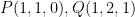$P(1,1,0),Q(1,2,1)$ and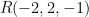$R( - 2,2, - 1)$.
 Solution: 10
Let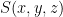$S(x,y,z)$ be any arbitrary point in the plane whose equation we wish to determine:Since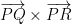$\overrightarrow {PQ} \times \overrightarrow {PR}$ will be perpendicular to this plane, we must have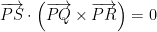$\overrightarrow {PS} \cdot \left( {\overrightarrow {PQ} \times \overrightarrow {PR} } \right) = 0$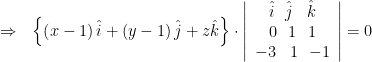$\Rightarrow \,\,\,\,\left\{ {\left( {x - 1} \right)\hat i + \left( {y - 1} \right)\hat j + z\hat k} \right\} \cdot \left| {\begin{array}{*{20}{c}} {\hat i}\,\,\,\,{\hat j}\,\,\,\,\,{\hat k}\\ 0\,\,\,\,1\,\,\,\,1\\ { - 3}\,\,\,\,\,1\,\,\,\,{ - 1} \end{array}} \right| = 0$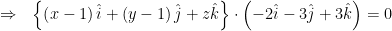$\Rightarrow \,\,\,\, \left\{ {\left( {x - 1} \right)\hat i + \left( {y - 1} \right)\hat j + z\hat k} \right\} \cdot \left( { - 2\hat i - 3\hat j + 3\hat k} \right) = 0$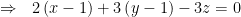$\Rightarrow \,\,\,\, 2\left( {x - 1} \right) + 3\left( {y - 1} \right) - 3z = 0$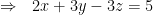$\Rightarrow \,\,\,\, 2x + 3y - 3z = 5$
We could have proceeded alternatively as follows: using the result of the last example, any arbitrary plane through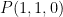$P(1,1,0)$ will be of the form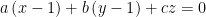$a\left( {x - 1} \right) + b\left( {y - 1} \right) + cz = 0$$\Rightarrow \,\,\,\,{\lambda _1}\left( {x - 1} \right) + {\lambda _2}\left( {y - 1} \right) + z = 0 \,\,\,\, ;{\lambda _1} = \dfrac{a}{c},\,{\lambda _2} = \dfrac{b}{c}$
If this passes through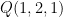$Q(1,2,1)$ and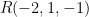$R( - 2,1, - 1)$, we have${\lambda _2} + 1 = 0$
and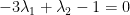$- 3{\lambda _1} + {\lambda _2} - 1 = 0$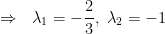$\Rightarrow \,\,\,\, {\lambda _1} = - \dfrac{2}{3},\,\,{\lambda _2} = - 1$
Thus the equation of the plane is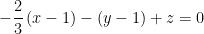$- \dfrac{2}{3}\left( {x - 1} \right) - \left( {y - 1} \right) + z = 0$$\Rightarrow \,\,\,\, 2\left( {x - 1} \right) + 3\left( {y - 1} \right) - 3z = 0$$\Rightarrow \,\,\,\, 2x + 3y - 3z = 5$
 Example: 11
 Find the equation of the plane intercepting lengths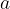$a$,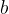$b$ and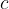$c$ on the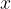$x$-,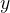$y$- and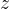$z$-axis respectively.
 Solution: 11
The plane passes through the points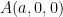$A(a,0,0)$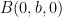$B(0,b,0)$ and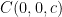$C(0,0,c)$. For any variable point$S(x,y,z)$ in this plane, we have (as discussed in the previous section),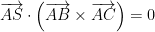$\overrightarrow {AS} \cdot \left( {\overrightarrow {AB} \times \overrightarrow {AC} } \right) = 0$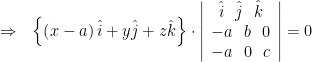$\Rightarrow \,\,\,\, \left\{ {\left( {x - a} \right)\hat i + y\hat j + z\hat k} \right\} \cdot \left| {\begin{array}{*{20}{c}} {\hat i}\,\,\,\,{\hat j}\,\,\,\,{\hat k}\\ { - a}\,\,\,\,b\,\,\,\,0\\ { - a}\,\,\,\,0\,\,\,\,c \end{array}} \right| = 0$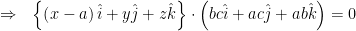$\Rightarrow \,\,\,\, \left\{ {\left( {x - a} \right)\hat i + y\hat j + z\hat k} \right\} \cdot \left( {bc\hat i + ac\hat j + ab\hat k} \right) = 0$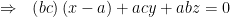$\Rightarrow \,\,\,\, \left( {bc} \right)\left( {x - a} \right) + acy + abz = 0$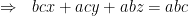$\Rightarrow \,\,\,\, bcx + acy + abz = abc$$\Rightarrow\,\,\,\,\,{\dfrac{x}{a} + \dfrac{y}{b} + \dfrac{z}{c} =1 }$
This general equation has the same form as the equation of the line in intercept form; which further proves the analogy between the formulae in two and in three dimensions.
 Example: 12
 A plane is at a distance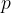$p$ from the origin and the direction cosines of the (outward) normal to it are$l$,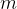$m$,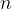$n$. Find its equation.
 Solution: 12
The unit vector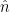$\hat n$ normal to the plane is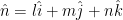$\hat n = l\hat i + m\hat j + n\hat k$
For any point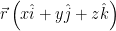$\vec r\left( {x\hat i + y\hat j + z\hat k} \right)$ in the plane, we have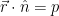$\vec r \cdot \hat n = p$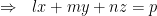$\Rightarrow \,\,\,\,lx + my + nz = p$
This is the required equation; it is called the normal form of the plane’s equation. As an exercise, convert the general equation of the plane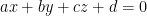$ax + by + cz + d = 0$
into normal form.